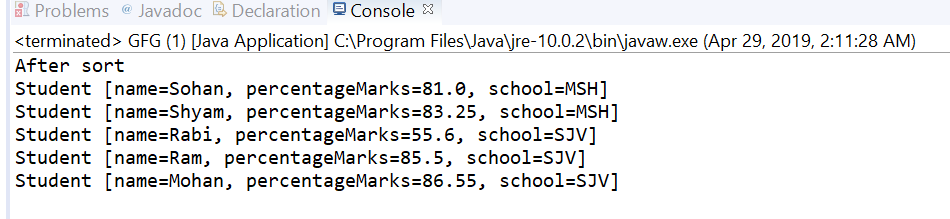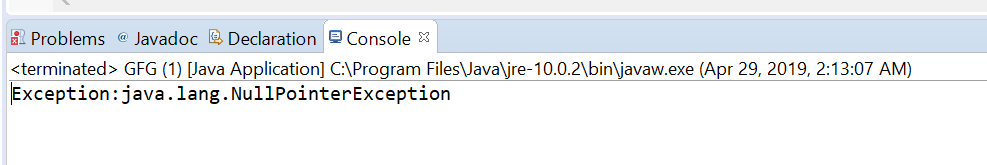# Comparator thenComparingDouble() method in Java with examples

The thenComparingDouble(java.util.function.ToDoubleFunction) method of Comparator Interface in Java returns a lexicographic-order comparator with a function that extracts a double sort key. This method is applied after comparing method if you want to apply another comparing for those values which are equal in the comparing method.

Syntax:

```default Comparator <T> thenComparingDouble(
ToDoubleFunction <T> keyExtractor)
```

Parameters: This method accepts keyExtractor which is the function used to extract the Double sort key.

Return value: This method returns a lexicographic-order comparator composed of this and then the Double sort key.

Exception: This method throws NullPointerException if the argument is null.

Below programs illustrate thenComparingDouble(java.util.function.ToDoubleFunction) method:
Program 1:

 `// Java program to demonstrate Comparator ` `// thenComparingDouble(ToDoubleFunction) method ` ` `  `import` `java.util.Arrays; ` `import` `java.util.Collections; ` `import` `java.util.Comparator; ` `import` `java.util.List; ` ` `  `public` `class` `GFG { ` ` `  `    ``public` `static` `void` `main(String[] args) ` `    ``{ ` `        ``List list = getStudentList(); ` ` `  `        ``Comparator comparator ` `            ``= Comparator ` `                  ``.comparing(Student::getSchool) ` `                  ``.thenComparingDouble(Student::getpercentageMarks); ` ` `  `        ``Collections.sort(list, comparator); ` `        ``System.out.println(``"After sort"``); ` `        ``list.forEach(s -> System.out.println(s)); ` `    ``} ` ` `  `    ``public` `static` `List getStudentList() ` `    ``{ ` ` `  `        ``Student s1 = ``new` `Student(``"Ram"``, ``85.5``, ``"SJV"``); ` `        ``Student s2 = ``new` `Student(``"Shyam"``, ``83.25``, ``"MSH"``); ` `        ``Student s3 = ``new` `Student(``"Mohan"``, ``86.55``, ``"SJV"``); ` `        ``Student s4 = ``new` `Student(``"Sohan"``, ``81.00``, ``"MSH"``); ` `        ``Student s5 = ``new` `Student(``"Rabi"``, ``55.6``, ``"SJV"``); ` `        ``List list = Arrays.asList(s1, s2, s3, s4, s5); ` ` `  `        ``return` `list; ` `    ``} ` `} ` ` `  `class` `Student { ` ` `  `    ``private` `String name; ` `    ``private` `double` `percentageMarks; ` `    ``private` `String school; ` ` `  `    ``public` `Student(String name, ` `                   ``double` `percentageMarks, ` `                   ``String school) ` `    ``{ ` `        ``this``.name = name; ` `        ``this``.percentageMarks = percentageMarks; ` `        ``this``.school = school; ` `    ``} ` ` `  `    ``public` `String getName() ` `    ``{ ` `        ``return` `name; ` `    ``} ` ` `  `    ``public` `void` `setName(String name) ` `    ``{ ` `        ``this``.name = name; ` `    ``} ` ` `  `    ``public` `double` `getpercentageMarks() ` `    ``{ ` `        ``return` `percentageMarks; ` `    ``} ` ` `  `    ``public` `void` `setpercentageMarks(``int` `percentageMarks) ` `    ``{ ` `        ``this``.percentageMarks = percentageMarks; ` `    ``} ` ` `  `    ``public` `String getSchool() ` `    ``{ ` `        ``return` `school; ` `    ``} ` ` `  `    ``public` `void` `setSchool(String school) ` `    ``{ ` `        ``this``.school = school; ` `    ``} ` ` `  `    ``@Override` `    ``public` `String toString() ` `    ``{ ` `        ``return` `"Student [name="` `+ name + ",  ` `            ``percentageMarks ` `            ``= " + percentageMarks  ` `              ``+ ``", school="` `+ school + ``"]"``; ` `    ``} ` `} `

The output printed on console of IDE is shown below.
Output:You can see in example first sorting is done on school wise and if the school is same then percentageMarks wise.

Program 2:

 `// Java program to demonstrate Comparator ` `// thenComparingDouble(ToDoubleFunction)  method ` ` `  `import` `java.util.Arrays; ` `import` `java.util.Comparator; ` `import` `java.util.List; ` ` `  `public` `class` `GFG { ` ` `  `    ``public` `static` `void` `main(String... args) ` `    ``{ ` ` `  `        ``List list ` `            ``= Arrays.asList(``1``, ``2``, ``3``, ``4``, ``5``, ``6``); ` ` `  `        ``try` `{ ` ` `  `            ``// apply thenComparingDouble ` `            ``Comparator.comparing(list::get) ` `                ``.thenComparingDouble(``null``); ` `        ``} ` `        ``catch` `(Exception e) { ` ` `  `            ``System.out.printf(``"Exception:"` `+ e); ` `        ``} ` `    ``} ` `} `

The output printed on console is shown below.
Output:Attention reader! Don’t stop learning now. Get hold of all the important DSA concepts with the DSA Self Paced Course at a student-friendly price and become industry ready.

My Personal Notes arrow_drop_upCheck out this Author's contributed articles.

If you like GeeksforGeeks and would like to contribute, you can also write an article using contribute.geeksforgeeks.org or mail your article to contribute@geeksforgeeks.org. See your article appearing on the GeeksforGeeks main page and help other Geeks.

Please Improve this article if you find anything incorrect by clicking on the "Improve Article" button below.

Article Tags :
Practice Tags :

Be the First to upvote.

Please write to us at contribute@geeksforgeeks.org to report any issue with the above content.